# ISEE Upper Level Quantitative : How to find the volume of a cone

## Example Questions

### Example Question #1 : Cones

The height of Cone B is three times that of Cone A. The radius of the base of Cone B is one-half the radius of the base of Cone A.

Which is the greater quantity?

(a) The volume of Cone A

(b) The volume of Cone B

(a) and (b) are equal.

(b) is greater.

It is impossible to tell from the information given.

(a) is greater.

(a) is greater.

Explanation:

Let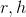be the radius and height of Cone A, respectively. Then the radius and height of Cone B are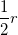and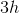, respectively.

(a) The volume of Cone A is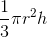.

(b) The volume of Cone B is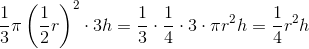.

Since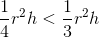, the cone in (a) has the greater volume.

### Example Question #3 : Volume Of A Cone

The volume of a cone whose height is three times the radius of its base is one cubic yard. Give its radius in inches.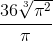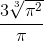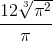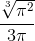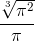Explanation:

The volume of a cone with base radiusand heightis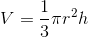The heightis three times this, or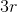. Therefore, the formula becomes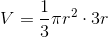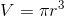Set this volume equal to one and solve for: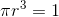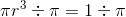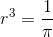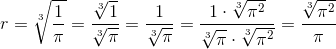This is the radius in yards; since the radius in inches is requested, multiply by 36.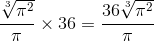### Example Question #2 : Cones

The height of a given cylinder is one half the height of a given cone. The radii of their bases are equal.

Which of the following is the greater quantity?

(a) The volume of the cone

(b) The volume of the cylinder

(b) is the greater quantity

It cannot be determined which of (a) and (b) is greater

(a) is the greater quantity

(a) and (b) are equal

(b) is the greater quantity

Explanation:

Callthe radius of the base of the cone and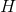the height of the cone. The cylinder will have bases of radiusand height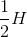.

In the formula for the volume of a cylinder, set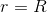and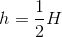: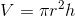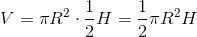In the formula for the volume of a cone, setand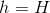: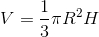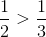, so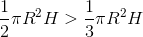,

meaning that the cylinder has the greater volume.

### Example Question #3 : Cones

The radius of the base of a given cone is three times that of each base of a given cylinder. The heights of the cone and the cylinder are equal.

Which of the following is the greater quantity?

(a) The volume of the cone

(b) The volume of the cylinder

(a) is the greater quantity

It cannot be determined which of (a) and (b) is greater

(b) is the greater quantity

(a) and (b) are equal

(a) is the greater quantity

Explanation:

If we letbe the radius of each base of the cylinder, then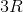is the radius of the base of the cone. We can letbe their common height.

In the formula for the volume of a cylinder, setand: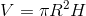In the formula for the volume of a cone, set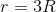and: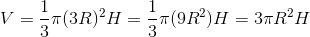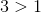, so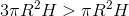. The cone has the greater volume.